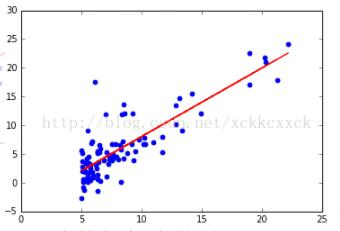# python实现简单的单变量线性回归方法

更新时间：2018年11月08日 11:45:29   作者：xckkcxxck我要评论

sklearn对机器学习方法封装的十分好，基本使用fit,predict,score，来训练，预测，评价模型，

```from pandas import DataFrame
from pandas import DataFrame
import pandas as pd
import matplotlib.pyplot as plt
from sklearn import datasets,linear_model

X=[]
Y=[]
with open("C:\\Users\\www\\ex1data1.txt","r") as f:  #读取txt文件。
for line in f:
p_tmp, E_tmp = [float(i) for i in line.split(',')]
X.append(p_tmp)
Y.append(E_tmp)
#'data=np.loadtxt('ex1data1.txt',delimiter=',')```
`# X=data`
`# Y=data `
```data=DataFrame(X,columns={'a'})
data['b']=b
X=DataFrame(X)

fig = plt.figure()
ax1 = fig.add_subplot(1,1,1)
plt.scatter(data['a'],data['b'])   #显示X，Y的散点图

def linear_model_main(X,Y,predict_value): #定义一个使用线性回归的函数
regr=linear_model.LinearRegression()
regr.fit(X,Y)  #训练模型
predict_output=regr.predict(predict_value) #预测
predictions={}        #用一个集合装以下元素
predictions['intercept']=regr.intercept_  #截距
predictions['codfficient']=regr.coef_   #斜率（参数）
predictions['predict_value']=predict_output  #预测值
return predictions

result = linear_model_main(X,Y,1500)    #调用函数
print(result['predict_value'])

def show_predict(X,Y):
regr=linear_model.LinearRegression()
regr.fit(X,Y)
plt.scatter(X,Y,color='blue')
plt.plot(X,regr.predict(X),color='red')

show_predict(X,Y)
```